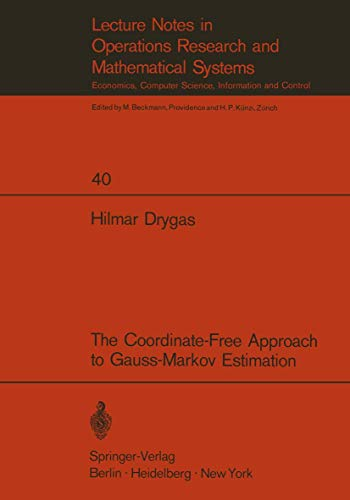Free Shipping in Australia
Proud to be B-Corp

# The Coordinate-Free Approach to Gauss-Markov Estimation H. Drygas\$203.89
Condition - New
Only 2 left

## The Coordinate-Free Approach to Gauss-Markov Estimation Summary

### The Coordinate-Free Approach to Gauss-Markov Estimation by H. Drygas

These notes originate from a couple of lectures which were given in the Econometric Workshop of the Center for Operations Research and Econometrics (CORE) at the Catholic University of Louvain. The participants of the seminars were recommended to read the first four chapters of Seber's book , but the exposition of the material went beyond Seber's exposition, if it seemed necessary. Coordinate-free methods are not new in Gauss-Markov estimation, besides Seber the work of Kolmogorov , SCheffe , Kruskal ,  and Malinvaud ,  should be mentioned. Malinvaud's approach however is a little different from that of the other authors, because his optimality criterion is based on the ellipsoid of c- centration. This criterion is however equivalent to the usual c- cept of minimal covariance-matrix and therefore the result must be the same in both cases. While the usual theory gives no indication how small the covariance-matrix can be made before the optimal es timator is computed, Malinvaud can show how small the ellipsoid of concentration can be made: it is at most equal to the intersection of the ellipssoid of concentration of the observed random vector and the linear space in which the (unknown) expectation value of the observed random vector is lying. This exposition is based on the observation, that in regression ~nalysis and related fields two conclusions are or should preferably be applied repeatedly.

Content.- 1. Justification of the coordinate-free approach.- 2. Vector-spaces.- a) Definition of a vector-space.- b) Inner products and semi-inner products.- c) Bases of a vector-space, orthogonal complement.- d) Linear functions, linear mappings and adjoint mappings.- e) Definition of set-operations in vector-spaces.- f) The Farkas' theorem.- g) Projections, generalized inverses and pseudo-inverses.- 3. Linear statistical models.- a) Definition of linear statistical models.- b) Least squares-estimators and Gauss-Markov estimators.- c) Supplements to least squares and Gauss-Markov estimation.- d) Examples:.- 1) Restrictions.- 2) The regression model.- 3) Aitken's formula.- 4) Schoenfeld's formula in the general regression model.- 5) Restrictions on the parameters.- e) The estimation of ?2.- f) Stepwise least squares and stepwise Gauss-Markov estimation.

NLS9783540053262
9783540053262
3540053263
The Coordinate-Free Approach to Gauss-Markov Estimation by H. Drygas
New
Paperback
Springer-Verlag Berlin and Heidelberg GmbH & Co. KG
1970-01-01
118
N/A
Book picture is for illustrative purposes only, actual binding, cover or edition may vary.
This is a new book - be the first to read this copy. With untouched pages and a perfect binding, your brand new copy is ready to be opened for the first time